The Volume of the Stella Octangula

The stellated octahedron is the only stellation of the octahedron. It was depicted by Leonardo da Vinci in Pacioli's book 'De Divina Proportione' in 1509.Leonardo da Vinci made several drawings of polyhedra for Luca Pacioli's book 'De divina proportione'. Here we can see an adaptation of the stellated octahedron (stella octangula).

A hundred years later was named 'Stella octangula' by Johannes Kepler.

It can be seen as the compound of two tetrahedra (one tetrahedron an its dual, a second tetrahedron). The eight vertices are the vertices of a cube.

As an stellation is an octahedron with tetrahedral pyramids on each face.

Then is very easy to calculate the volume of an stellated octahedron if we know the volume of an octahedron and the volume of a tetrahedron.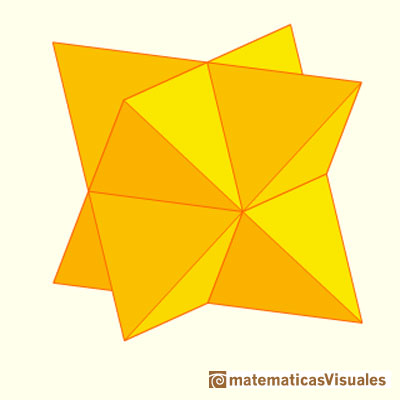Then the volume of an stellated octahedron of side length a is:

Now we are going to think a little more about duality.

The main elements of a polyhedron are vertices, edges and faces. For example, a tetrahedron has four vertices, six edges and four faces.

The dual (or reciprocal) of any polyhedron is one that has the same number of edges as the original which it is derived, but there is an interchange in the number of faces and vertices. (Wenninger)

For example, the cube and the octahedron are dual polyhedra. A cube has eight vertices and six faces and an octahedron has eight faces and six vertices. Both have twelve edges.

In the case of the tetrahedron, a tetrahedron has four vertices and four faces. Then, the dual of a tetrahedron is another tetrahedron. We say that the tetrahedron is self-dual.

Starting with a regular polyhedron, the dual can be constructed considering the incenter of each face and connecting this points with points in neighboring faces. These edges are the edges of the dual polyhedron.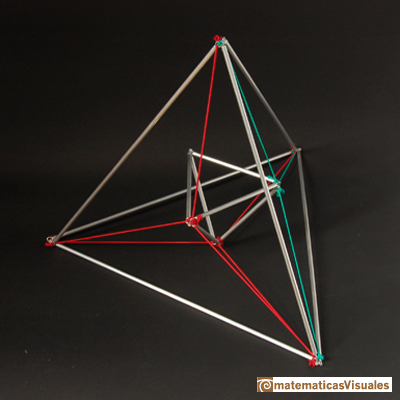What is the relative size of these two tetrahedra?

Another interesting way to construct the dual of a Platonic or Archimedean solid is to adjust the sizes in a way (reciprocation) that the respective edges become the perpendicular bisectors of the corresponding edges of its dual. Then a compound polyhedron is formed.

In the case of the tetrahedron, the tetrahedron with its dual becomes a compound of two tetrahedra: Kepler's Stella Octangula.

As we saw before, the solid common to the two solids is an octahedron.

These two tetrahedra are contained in a cube.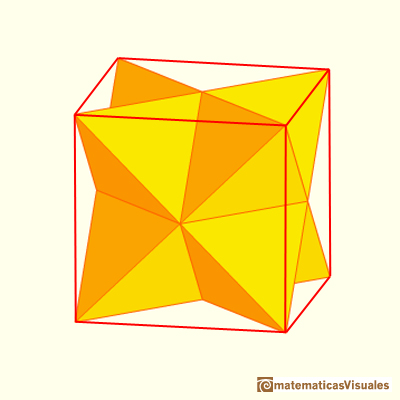The edges of the Stella Octangula are diagonals of the faces of the cube and meet in pairs at the vertices of the octahedron. (Cundy, p. 129)

We can consider that the Stella Octangula has eight faces that lie in the facial planes of an octahedron (Stella octangula is the stellation of an octahedron).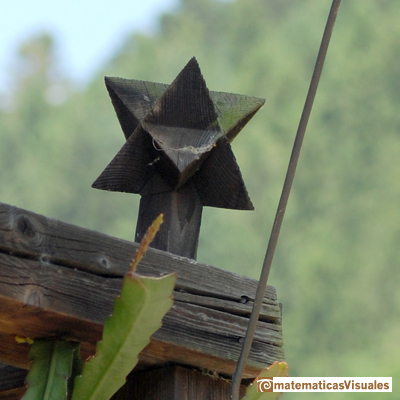Detail in a house at The Black Forest Open Air Museum (near Gutach, Germany)

REFERENCES

Hugo Steinhaus - Mathematical Snapshots - Oxford University Press - Third Edition (p. 197)
Magnus Wenninger - 'Polyhedron Models', Cambridge University Press.
Magnus Wenninger - 'Dual Models', Cambridge University Press.
Peter R. Cromwell - 'Polyhedra', Cambridge University Press, 1999.
H.Martin Cundy and A.P. Rollet, 'Mathematical Models', Oxford University Press, Second Edition, 1961 (p. 87).
W.W. Rouse Ball and H.S.M. Coxeter - 'Matematical Recreations & Essays', The MacMillan Company, 1947.
Luca Pacioli - De divina proportione - (La divina proporción) Ediciones Akal, 4ª edición, 2004. Spanish translation by Juan Calatrava.

MORE LINKSThe volume of an octahedron is four times the volume of a tetrahedron. It is easy to calculate and then we can get the volume of a tetrahedron.A cuboctahedron is an Archimedean solid. It can be seen as made by cutting off the corners of a cube.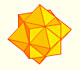The compound polyhedron of a cube and an octahedron is an stellated cuboctahedron.It is the same to say that the cuboctahedron is the solid common to the cube and the octahedron in this polyhedron.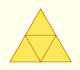The first drawing of a plane net of a regular tetrahedron was published by Dürer in his book 'Underweysung der Messung' ('Four Books of Measurement'), published in 1525 .We study different prisms and we can see how they develop into a plane net. Then we explain how to calculate the lateral surface area.Plane nets of prisms with a regular base with different side number cut by an oblique plane.We study different cylinders and we can see how they develop into a plane. Then we explain how to calculate the lateral surface area.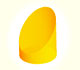We study different cylinders cut by an oblique plane. The section that we get is an ellipse.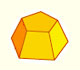Plane net of pyramids and pyramidal frustrum. How to calculate the lateral surface area.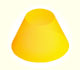Plane developments of cones and conical frustum. How to calculate the lateral surface area.Plane developments of cones cut by an oblique plane. The section is an ellipse.The first drawing of a plane net of a regular dodecahedron was published by Dürer in his book 'Underweysung der Messung' ('Four Books of Measurement'), published in 1525 .The twelve vertices of an icosahedron lie in three golden rectangles. Then we can calculate the volume of an icosahedronSome properties of this platonic solid and how it is related to the golden ratio. Constructing dodecahedra using different techniques.The truncated octahedron is an Archimedean solid. It has 8 regular hexagonal faces and 6 square faces. Its volume can be calculated knowing the volume of an octahedron.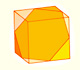We can cut in half a cube by a plane and get a section that is a regular hexagon. Using eight of this pieces we can made a truncated octahedron.Using eight half cubes we can make a truncated octahedron. The cube tesselate the space an so do the truncated octahedron. We can calculate the volume of a truncated octahedron.These polyhedra pack together to fill space, forming a 3 dimensional space tessellation or tilling.The truncated octahedron is an Archimedean solid. It has 8 regular hexagonal faces and 6 square faces. Its volume can be calculated knowing the volume of an octahedron.You can chamfer a cube and then you get a polyhedron similar (but not equal) to a truncated octahedron. You can get also a rhombic dodecahedron.Leonardo da Vinci made several drawings of polyhedra for Luca Pacioli's book 'De divina proportione'. Here we can see an adaptation of the truncated octahedron.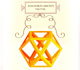Leonardo da Vinci made several drawings of polyhedra for Luca Pacioli's book 'De divina proportione'. Here we can see an adaptation of the cuboctahedron.Leonardo da Vinci made several drawings of polyhedra for Luca Pacioli's book 'De divina proportione'. Here we can see an adaptation of the dodecahedron.Leonardo da Vinci made several drawings of polyhedra for Luca Pacioli's book 'De divina proportione'. Here we can see an adaptation of the stellated octahedron (stella octangula).The truncated tetrahedron is an Archimedean solid made by 4 triangles and 4 hexagons.When you truncate a cube you get a truncated cube and a cuboctahedron. If you truncate an octahedron you get a truncated octahedron and a cuboctahedron.